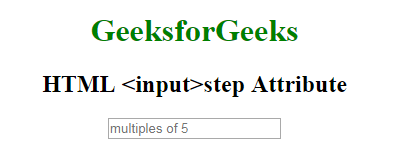Related Articles

# HTML | step Attribute

• Last Updated : 08 Sep, 2021

The HTML <input> step Attribute in HTML is used to set the discrete step size of the <input> element. The default stepping value for number inputs is 1.
Usage: It works with the following input types:

• number
• range
• date
• datetime-local
• month
• time
• week

Syntax:

Attention reader! Don’t stop learning now. Get hold of all the important HTML concepts with the Web Design for Beginners | HTML  course.

`<input step = "value"> `

Attribute Values: It contains a value i.e number which specifies the legal number interval for the number field. It has a default value which is 1.

Example: This Example illustrates the use of step attribute in <input> element.

## html

 ```<``html``>` `<``head``>``    ``<``title``>``      ``HTML input step Attribute``  ````` `<``body``>``  ``<``center``>``    ``<``h1` `style``=``"color: green;"``>``            ``GeeksforGeeks``        ``` `    ``<``h2``>``            ``HTML <``input``>step Attribute``        ``` `    ``<``input` `type``=``"number"``           ``name``=``"points"``           ``step``=``"5"``           ``placeholder``=``"multiples of 5"``>` `  ````` ``

Output:Supported Browsers: The browsers supported by <input> step Attribute are listed below: